## Medium Transmission Line:

For lines more than 100 km long, charging currents due to shunt admittance cannot be neglected. For lines in range 100 km to 250 km length, it is sufficiently accurate to lump all the line admittance at the receiving-end resulting in the equivalent diagram of Medium Transmission Line shown in Fig. 5.7. Starting from fundamental circuit equations, it is fairly straightforward to write the Medium Transmission Line equations in the ABCD constant form given below: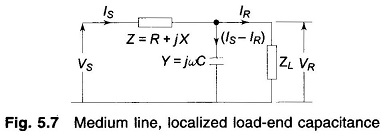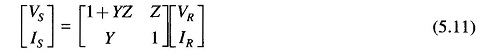### Nominal-T Representation:

If all the shunt capacitance is lumped at the middle of the line, it leads to the nominal-T circuit shown in Fig. 5.8.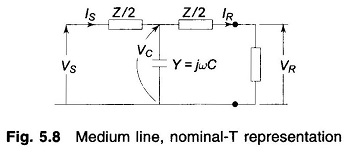For the nominal-T circuit, the following circuit equations can be written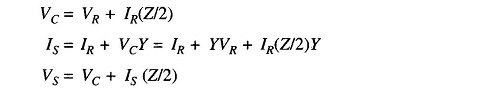Substituting for VC and IS in the last equation, we get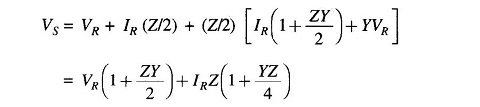Rearranging the results, we get the following equations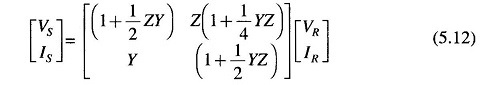### Nominal- π Representation:

In this method the total line capacitance is divided into two equal parts which are lumped at the sending- and receiving-ends resulting in the nominal- π representation as shown in Fig. 5.9.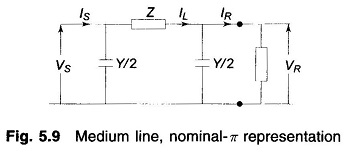From Fig. 5.9, we have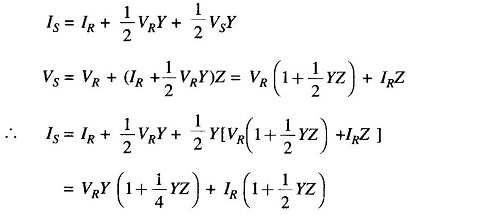Finally, we have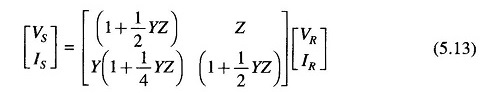It should be noted that nominal-T and nominal- π with the above constants are not equivalent to each other. The reader should verify this fact by applying star-delta transformation to either one.

Scroll to Top